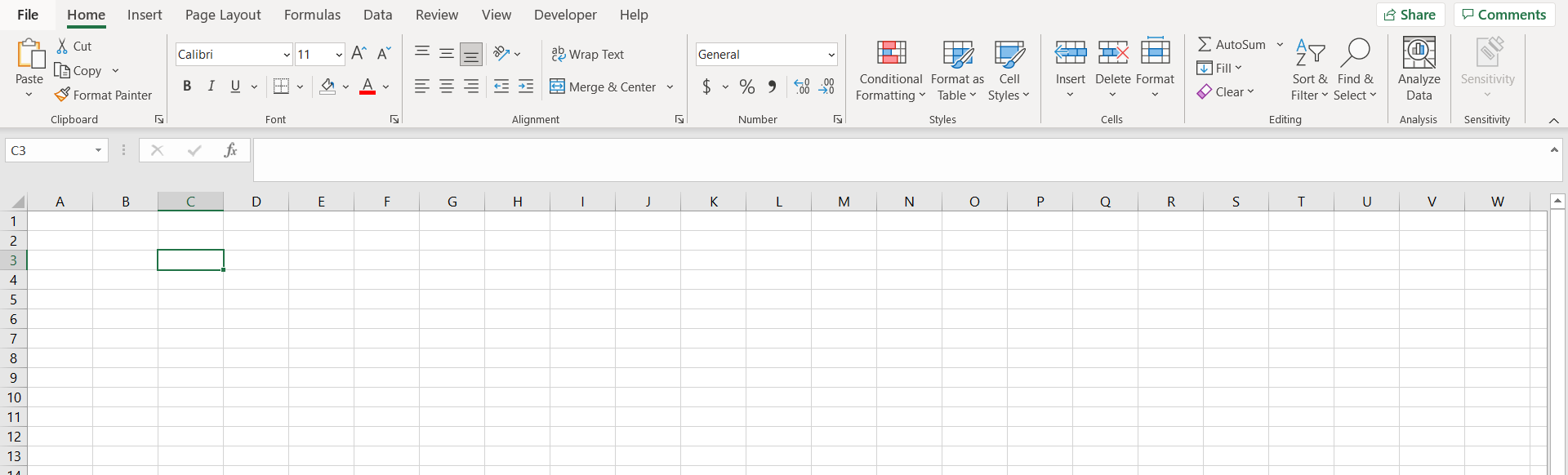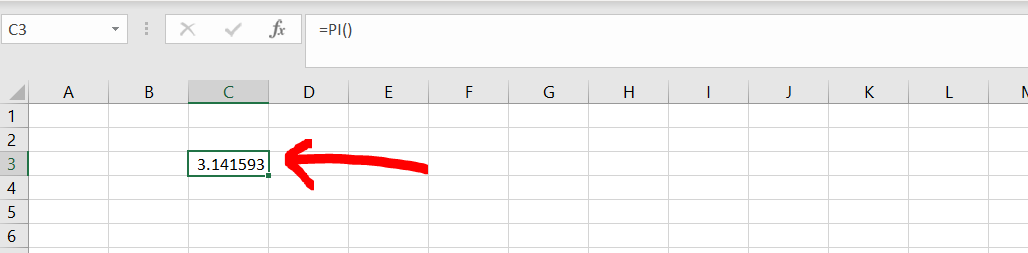# How to do Pi in ExcelMicrosoft Excel offers a very interesting way to do pi. We can perform the below mentioned way to a formula to do pi excel:

### Step 1 – Excel with a blank worksheet– Open the desired Excel workbook, and make sure there is a blank worksheet in it.

### Step 2 – Using the Pi formula– Now as shown in the image above, enter the formula “=PI()”.

### Step 3 – Getting the value of pi– Now if we hit enter, we will be able to see the value of pie, as shown in the image above.